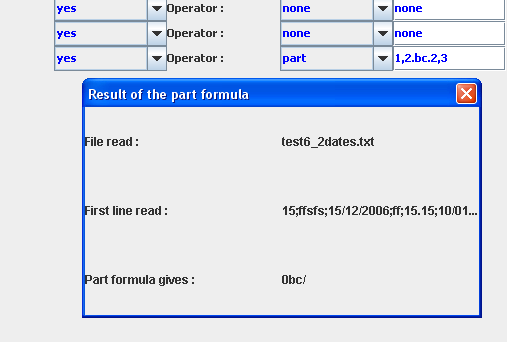Benetl is a free ETL tool an ETL to easily manage csv/txt/xls/json files51210

# 12. Part operator syntax

Part operator syntax:

This is a special operator for fields that is called: "part".

This operator allows you to:

• retrieve a sub-string from the value of the field using it, with a regular expression
• retrieve a sub-string from the datasource file name
• add strings to the value of the field
• use values from previously parsed fields
• combine mathematics functions together with values and/or fields values
• The interface (GUI) of Benetl provides a function called "part formula viewer".

It helps you to define and test your "part formula" by giving you the result according to the datasource line to be read.

Click on the "part" formula and then press enter. It will then open the part formula viewer.The "part" operator has a special syntax:

In order to retrieve a sub-string from the value of the current field, you can use the word "regex".

Example: regex(([0-9]+)[\W\w]+)

Do not use "." character in the regex syntax because the "." character is used to concat sub-string here.
Instead of "." please use "\W\w" which can retrieve any alphanum character.

In order to retrieve a sub-string from the filename, you can use the word "filename".

Example: filename(19,21)

First number is the start position and second number is the end position of the sub-string from datasource file name.

In order to add a string to the value of the current field you can use "." character.

Example: . bonjour

This will add " bonjour" to the value of the field of each lines of the datasource file read.

If you need to use a value from a previously parsed field you can use the word "field".
This word is followed by the number of the field.

Example: 01/.field3./.field17

In this example we are adding "01/" to the value of the field number 3, then we add "/" and the value of the field number 17. The first field is "field1", it is the first field you can find in "control_file.xml" of your EtlTool.

This example was retrieving a date: field3 was month and field17 was a year.

A much more complex example is combining several words of "part" syntax:

Example:

filename(19,21)./.filename(17,19)./.filename(13,17). .regex((([0-9]+))[\W\w]+).:00:00

This example was retrieving the day (position 19 to 21), the month (position 17 to 19), the year (position 13 to 17) from the datasource filename. It is also retrieving hours from the current field value, with a regular expression. It is also adding ":00:00" wich is meaning 0 minute 0 second.

All of this is retrieving a complete date: 29/12/2007 3:00:00, which is corresponding to a french date format.

line_number will add the number of the line read to the formula.

With interval it will possible to add or remove a timestamp interval from your dates.
It has to be used with a "date" field. The interval function must be in the last position of a "part formula" .

Examples:

— interval ’1 month’

+interval ’2 month’

Note: no spaces between +/- interval and ’x month’.

• uppercase
• uppercase(field) or uppercase
• lowercase
• lowercase(field) or lowercase

Benetl 2.2 supports 23 mathematics functions you can use and combine into a same "." part of your "part formula":

• inv
• inv(field) or inv
• abs
• abs(field) or abs
• min
• min(field1;field2) or min(field1;value) or min(value;field1)
• max
• max(field1;field2) or max(field1;value) or max(value;field1)
• avg
• avg(field1;field2) or avg(field1;value) or avg(value;field1)
• plus
• plus(field1;field2) or plus(field1;value) or plus(value;field1)
• minus
• minus(field1;field2) or minus(field1;value) or minus(value;field1)
• divide
• divide(field1;field2) or divide(field1;value) or divide(value;field1)
• multiply
• multiply(field1;field2) or multiply(field1;value) or multiply(value;field1)
• dist
• dist(field1;field2) or dist(field1;value) or dist(value;field1)
• round
• round(field1) or round(value)
• ceil
• ceil(field1) or ceil(value)
• floor
• floor(field1) or floor(value)
• square
• square(field1) or square(value)
• pow
• pow(field1;field2) or pow(field1;value) or pow(value;field2)
• exp
• exp(field1) or exp(value)
• log
• log(field1) or log(value)
• asin
• asin(field1) or asin(value)
• sin
• sin(field1) or sin(value)
• acos
• acos(field1) or acos(value)
• cos
• cos(field1) or cos(value)
• atan
• atan(field1) or atan(value)
• tan
• tan(field1) or tan(value)

Examples of combination:

avg(max(field3;10,5);field3)

min(plus(3,5;field2);15)

Important:
for these functions the argument separator is ";" and "," is used for floats. French localized users must use "," as float here because "." is used as a concat operator in "part formula".

Important:
if you don’t mention a field in functionnalities with unique argument, then the current field is used.

Important:
these mathematics functionalities (inv, abs, min, max, avg, plus, minus, divide, multiply, dist, round, ceil, floor, square, pow, exp, log, asin, sin, acos, cos, atan, tan) can not be used with "regex", "filename", "interval", "lowercase", "uppercase", "line_number" inside a same part "." of the "part formula".

Benetl 2.7 adds 4 new functions:

• replace
• replace(value1;value2) since version 2.9 separator is ";" for replace function
• today
• today()
• tomorrow
• tomorrow()
• yesterday
• yesterday()

Benetl 2.8 adds a new function:

• substr
• substr(field1;value1;value2)

Example of substr use:

substr(field2;0;2)

Benetl 3.5 adds a new function:

• if
• if(fieldX==4;ok;false)

if(fieldX>=fieldY;ok;false)

if(fieldX<=6.5;ok;false)

if(fieldX==5;ok;false)

if(fieldX contains ben;ben;fieldX)

Benetl 4.1 adds to this function:

if(fieldX>fieldY;ok;false)

if(fieldX<6.5;ok;false)

The first argument is the condition. The second argument is used if the condition is true. The third argument is used if the condition is false. You can use "if" function with the mathematics functions described above.